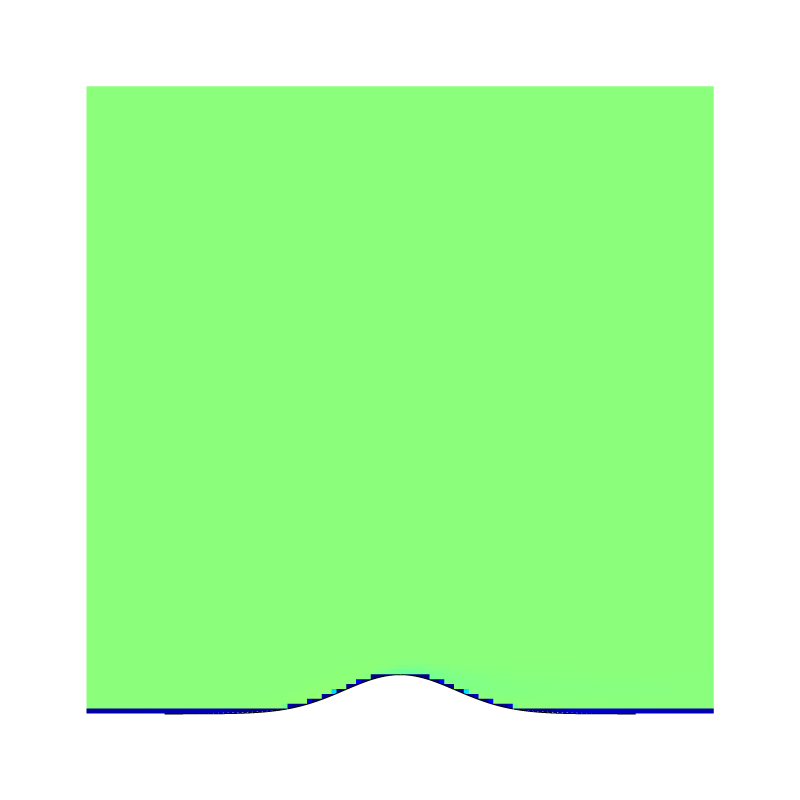# sandbox/Antoonvh/embed-freeslip.c

## Flow over an obstacle

On this page we embed a free slip mountain into a domain that is filled with an viscous fluid. The free-slip condition is not free of rotation along a curved boundary. Therefore, simple potential flow theory does not apply and we solve the equations for fluid motion.

#include "grid/multigrid.h"
#include "embed.h"
#include "navier-stokes/centered.h"
#include "view.h"
#define BVIEW 1
#include "particles.h"

We set a free-slip boundary for the x and y components.

u.n[embed] = neumann (0);
u.t[embed] = neumann (0);

u.n[left] = dirichlet(min(t, 1.)*fm.x[]);
u.n[right] = neumann(0);
p[right] = dirichlet(0);
pf[right] = dirichlet(0);

face vector muc[];
int main(){
L0 = 8.;
for (N = 64; N <= 256; N *= 2){
init_grid(N);
run();
}
}

event properties(i++){
foreach_face()
muc.x[] = fm.x[] / 100.;
boundary((scalar*){muc});
}

We use particles to check if the flow does not penetrate the embedded boundary.

event init (t = 0){
init_particles_2D_square_grid(5, 1, 0.52, 1);
mu = muc;
scalar phi[];
foreach_vertex()
phi[] = y - 0.5*exp(-sq(x - L0/2));
fractions(phi, cs, fs);
boundary(all);
output_ppm(cs, n = 512, file = "cs.png", min = 0, max = 1);
}

To help the accurate evaluation of the viscous term, we enforce the time step according to a limit cell-Peclet number.

double PECLET = 0.5;
event stability(i++){
DT = 1.;
foreach_face(){
if (cs[] > 0.005 && fm.x[] > 0.005)
DT = min(DT, PECLET*sq(Delta)*cs[]/muc.x[]);
}
}

We generate a movie displaying the flow via u_x and the tracers, these do not penetrate the boundary.

The particles follow the flow along the boundary

event mover(t += 0.05){
if (N == 128){
clear();
view(tx = -0.5, ty = -0.5);
draw_vof("cs", filled = -1, fc = {1, 1, 1});
squares("u.x", min = 0, max = 2);
scatter(loc);
draw_vof("cs", "fs");
save("movie.mp4");
}
}

We also check if Bernoulli’s law is statisfied.

event stop(t = 10){
char fname;
sprintf(fname, "data%d", N);
FILE * fp = fopen(fname, "w");
foreach(){
if (y < L0/4 && fabs(x - L0/2.) < L0/4 && cm[] > 0.1)
fprintf(fp, "%g\t%g\n", p[], sq(u.x[]) + sq(u.y[]));
}
set output 'plot.png'
set term pngcairo
set xlabel 'Modified pressure'
set ylabel 'K'
set size square
set grid
set key outside
plot 'data256' t 'N = 256', 'data128' t 'N = 128', 'data64' t 'N = 64'There is an anti colleration between K and p … (script)

De deviation from the perfect anti correlation becomes less pronounced when a smaller value for the viscosity is used (not shown). Vorticity may diffuse into the domain along a curved free-slip boundary. As such, we check for the presence of vorticity via the entrophy.

  scalar omega[];
vorticity(u, omega);
double Omega = 0;
foreach()
Omega += sq(omega[])*sq(Delta)*cm[];
static FILE * fpo = fopen ("omega", "w");
fprintf(fpo, "%d\t%g\n", N, Omega);
set output 'plot2.png'
set term pngcairo
set logscale x 2
set xr [32:512]
set xlabel 'N'
set ylabel 'Omega'
set size square
set grid
set key off
plot 'omega' u 1:2 pt 5There is a small resolution dependence on the diagnosed enstrophy (script)

We check were in the domain the vorticity exists.

  if (N == 128){
clear();
view(tx = -0.5, ty = -0.5);
draw_vof("cs", filled = -1, fc = {1, 1, 1});
squares("omega");
draw_vof("cs", "fs");
save("vorticity.png");
}
}

We can observe that indeed vorticty diffuses into the domain. Also note that the sign of the boundary curvature dictates the sign of the vorticity that diffuses into the domain.A wake structure forms at the lee side of the free slip obstacle

A true eye-opener.